Tamilnadu State Board New Syllabus Samacheer Kalvi 11th Physics Guide Pdf Chapter 9 Kinetic Theory of Gases Text Book Back Questions and Answers, Notes.

## Tamilnadu Samacheer Kalvi 11th Physics Solutions Chapter 9 Kinetic Theory of Gases

### 11th Physics Guide Kinetic Theory of Gases Book Back Questions and AnswersI. Multiple choice questions:

Question 1.
A particle of mass m is moving with speed u in a direction which makes 60° with respect to x-axis. It undergoes elastic collision with the wall. What is the change in momentum in x and y direction?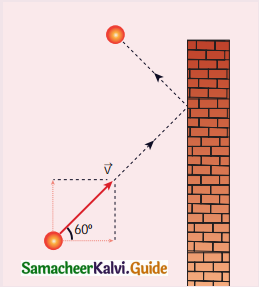(a) ∆px = – mu, ∆py = 0
(b) ∆px = – 2mu, ∆py = 0
(c) ∆px = 0, ∆py = mu
(d) ∆px = mu, ∆py = 0
(a) ∆px = – mu, ∆py = 0

Hint: As it moves with respect to X axis
∆px = – mu
∆py = 0

Question 2.
A sample of ideal gas is at equilibrium. Which of the following quantity is zero?
(a) rms speed
(b) average speed
(c) average velocity
(d) most probable speed
(c) average velocity

Hint:
vav = 1.6$$\sqrt{\frac{k \mathrm{~T}}{m}}$$
In equilibrium temperature T = 0Question 3.
An ideal gas is maintained at constant pressure. If the temperature of an ideal gas increases from 100K to 1000K then the rms speed of the gas molecules:
(a) increases by 5 times
(b) increases by 10 times
(c) remains same
(d) increases by 7 times
(b) increases by 10 times

Hint:
vrms = 1.73$$\sqrt{\frac{k \mathrm{~T}}{m}}$$
vrms ∝ ∆T
∆T is increased by 10 times.
∴ rms speed is increased by 10 times.

Question 4.
Two identically sized rooms A and B are connected by an open door. If the room A is air conditioned such that its temperature is 4° lesser than room B, which room has more air in it?
(a) Room A
(b) Room B .
(c) Both room has same air
(d) Cannot be determined
(a) Room A

Hint:
As temperature of room A is less than that of room B evidently, Room A has more air in it.Question 5.
The average translational kinetic energy of gas molecules depends on:
(a) number of moles and T
(b) only on T
(c) P and T
(d) P only
(a) number of moles and T

Hint: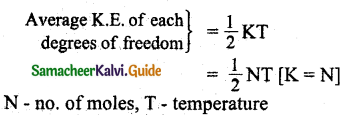Question 6.
If the internal energy of an ideal gas U and volume V are doubled then the pressure:
(a) doubles
(b) remains same
(c) halves
(b) remains same

Hint:
∆Q = ∆U + ∆W
∆U = ∆Q – ∆W

Question 7.
The ratio γ = $$\frac{\mathrm{C}_{\mathrm{P}}}{\mathrm{C}_{\mathrm{V}}}$$ for a gas mixture consisting of 8 g of helium and 16 g of oxygen is:
(a) 23/15
(b) 15/23
(c) 27/11
(d) 17/27
(a) 23/15

Hint:
Number of moles of helium n = $$\frac { 8 }{ 4 }$$ = 2
Number of moles of Oxygen n’ = $$\frac { 16 }{ 32 }$$ = $$\frac { 1 }{ 2 }$$
For monoatomic helium gas f = 3
Cv = $$\frac { f }{ 2 }$$R = $$\frac { 3 }{ 2 }$$R
For diatomic oxygen gas f = 5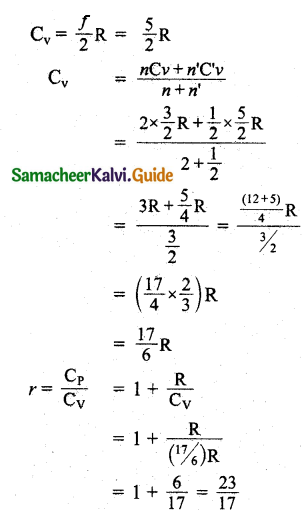Question 8.
A container has one mole of monoatomic ideal gas. Each molecule has f degrees of freedom. What is the ratio of γ = $$\frac{\mathrm{C}_{\mathrm{P}}}{\mathrm{C}_{\mathrm{V}}}$$?
(a) f
(b) $$\frac { f }{ 2 }$$
(c) $$\frac { f }{ f + 2 }$$
(d) $$\frac { f+2 }{ f }$$
(d) $$\frac { f+2 }{ f }$$

Question 9.
If the temperature and pressure of a gas is doubled the mean free’ path of the gas molecules:
(a) remains same
(b) doubled
(c) tripled
(a) remains same

Hint:
Mean free path is independent of temperature of pressure.Question 10.
Which of the following shows the correct relationship between the pressure and density of an ideal gas at constant temperature?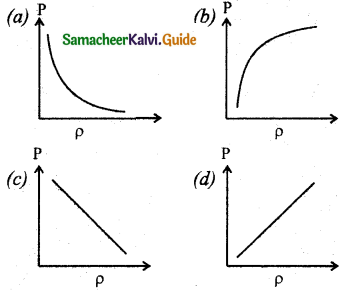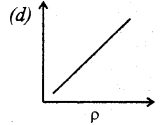Question 11.
A sample of gas consists of μ1 moles of monoatomic molecules, μ2 moles of diatomic molecules and μ3 moles of linear triatomic molecules. The gas is kept at high temperature. What is the total number of degrees of freedom?
(a) [3μ1 + 7(μ2 + μ3)] NA
(b) [3μ1 + 7μ2 + 6μ3] NA
(c) [7μ1 + 3(μ23)] NA
(d) [3μ1 + 6(μ2 + μ3)] NA
(a) [3μ1 + 7(μ2 + μ3)] NA

Hint:
For monoatomic molecule no. of degrees freedom = 3
For diatomic molecule no. of degrees of freedom = 5
For triatomic molecule no. of degrees of freedom = 7
Total = [3μ1 + 7(μ2 + μ3) ]NA

Question 12.
If sp and sv denote the specific heats of nitrogen gas per unit mass at constant pressure and constant volume respectively, then: (JEE 2007)
(a) sp and sv = 28R
(b) sp and sv = R/28
(c) sp and sv = R/14
(d) sp and sv = R
(b) sp and sv = R/28

Hint:
Cp – Cv = R
For diatomic gas N2 no. of degrees of freedom = 5
Sp – sv = R/28

Question 13.
Which of the following gases will have least rms speed at a given temperature?
(a) Hydrogen
(b) Nitrogen
(c) Oxygen
(d) Carbon dioxide
(d) Carbon dioxide

Hint:
vrms = 1.73$$\sqrt{\frac{k \mathrm{~T}}{m}}$$Question 14.
For a given gas molecule at a fixed temperature, the area under the Maxwell- Boltzmann distribution curve is equal to:
(a) $$\frac { PV }{ kT }$$
(b) $$\frac { kT }{ PV }$$
(c) $$\frac { P }{ NkT }$$
(d) PV
(a) $$\frac { PV }{ kT }$$

Hint:
The area under the graph will give total number of gas molecules in the system.
n = $$\frac { PV }{ RT }$$ R = k
n = $$\frac { PV }{ kT }$$

Question 15.
The following graph represents the pressure versus number density for ideal gas at two different temperatures T1 and T2. The graph implies: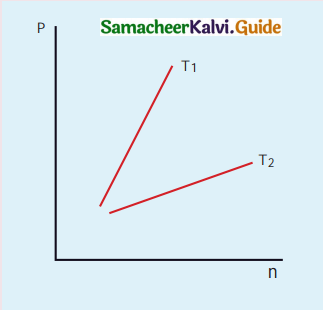(a) T1 = T2
(b) T1 > T2
(c) T1 < T2
(d) Cannot be determined
(b) T1 > T2

II. Short Answer Questions:

Question 1.
What is the microscopic origin of pressure? kinetic energy and pressure?
The microscopic origin of pressure was proposed by considering a thermodynamic system as a collection of molecules. By the kinetic theory of gases, the pressure is linked to the velocity of molecules (v) and number density ($$\frac {N}{ V }$$)
p = $$\frac { 1 }{ 3 }$$$$\frac { N }{ V }$$mv²
Where v – velocity of molecular
$$\frac { N }{ V }$$ – number density

Question 2.
What is the microscopic origin of T is uniformly distributed to all degrees of temperature?
The average K.E per molecule $$\overline{\mathrm{KE}}=\epsilon=\frac{3}{2} k \mathrm{T}$$
The equation implies that the temperature of a gas is a measure of the average translational K.E. per molecule of the gas.Question 3.
Why moon has no atmosphere?
The escape speed of gases on the surface of Moon is much less than the root mean square speeds of gases due to low gravity. Hence all molecules of the gases escape from the surface of the Moon easily.

Question 4.
Write the expression for rms speed, average speed and most probable speed of a gas molecule.
(i) The root mean square speed (rms):
vrms = $$\sqrt{\frac{3 R T}{M}}$$

(ii) Average speed:
$$\overline{v}$$ = 1.60$$\sqrt{\frac{k \mathrm{~T}}{m}}$$

(iii) Most probable speed:
vmp = 1.41$$\sqrt{\frac{k \mathrm{~T}}{m}}$$

Question 5.
What is the relation between the average kinetic energy and pressure?
P = $$\frac {2}{ 3 }$$$$\overline{KE}$$

Question 6.
Define the term degrees of freedom.
The minimum number of independent coordinates needed to specify the position and configuration of a thermo-dynamical system in space is called the degree of freedom of the system.Question 7.
State the law of equipartition of energy.
According to kinetic theory, the average kinetic energy of a system of molecules in thermal equilibrium at temperature T is uniformly distributed to all degrees of freedom (x or y or z directions of motion) so that each degree of freedom will get $$\frac {1}{ 2 }$$ kT of energy. This is called law of equipartition of energy.

Question 8.
Define mean free path and write down its expression.
Average distance travelled by the molecule between collisions is called mean free path (λ).
λ = $$\frac{1}{\sqrt{2} n \pi d^{2}}$$

Question 9.
Deduce Charle’s law based on kinetic theory.
PV = $$\frac {2}{ 3 }$$U
For a fixed pressure, the volume of the gas is proportional to internal energy of the gas.
(or)
Average kinetic energy is directly proportional to absolute temperature. It is implied that,
V ∝ T
(or) $$\frac {V}{ T }$$ = constant.

Question 10.
Deduce Boyle’s law based on kinetic theory.
We know that,
PV = $$\frac {2}{ 3 }$$U
But the internal energy of an ideal gas is equal to N times the average kinetic energy (∈) of each molecule.
U = N∈
For a fixed temperature, the average translational kinetic energy ∈ will remain constant. It implies that,
PV = $$\frac {2}{ 3 }$$N∈
Thus, PV = constantQuestion 11.
Deduce Avogadro’s law based on kinetic theory.
Avogadro’s law states that, at constant temperature and pressure, equal volumes of all gases contain the same number of molecules. For two different gases at the same temperature and pressure, according to kinetic theory of gases,
From equation,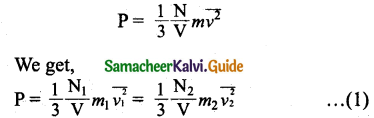where $$\overline{v_{1}^{2}}$$ and $$\overline{v_{2}^{2}}$$ are the mean square speed for two gases and N1 and N2 are the number of gas molecules in two different gases.

At the same temperature, the average kinetic energy per molecule is the same for two gases.
$$\frac {1}{ 2 }$$m1$$\overline{v_{1}^{2}}$$ = $$\frac {1}{ 2 }$$m2$$\overline{v_{2}^{2}}$$ … (2)
Dividing the equation (1) by (2) we get, N1 = N2.
This is Avogadro’s law. It is sometimes referred to as Avogadro’s hypothesis or Avogadro’s Principle.

Question 12.
List the factors affecting the mean free path.

1. Mean free path increases with increasing temperature. As the temperature increases, the average speed of each molecule will increase.
2. It is the reason why the smell of hot sizzling food reaches several metre away than smell of cold food.
3. The mean free path increases with decreasing pressure of the gas and diameter of the gas molecules.

Question 13.
What is the reason for the Brownian motion?
According to kinetic theory, any particle suspended in a liquid or gas is continuously bombarded from all directions so that the mean free path is almost negligible. This leads to the motion of the particles in a random and zig-zag manner.III. Long Answer Questions:

Question 1.
Write down the postulates of the kinetic theory of gases.

1. All the molecules of a gas are identical, elastic spheres.
2. The molecules of different gases are different.
3. The number of molecules in a gas is very large and the average separation between them is larger than size of the gas molecules.
4. The molecules of a gas are in a state of continuous random motion.
5. The molecules collide with one another and also with the walls of the container.
6. These collisions are perfectly elastic so that there is no loss of kinetic energy during collisions.
7. Between two successive collisions, a molecule moves with uniform velocity.
8. The molecules do not exert any force of attraction or repulsion on each other except during collision.
9. The molecules do not possess any potential energy and the energy is wholly kinetic.
10. The collisions are instantaneous. The time spent by a molecule in each collision is very small compared to the time elapsed between two consecutive collisions.
11. These molecules obey Newton’s laws of motion even though they move randomly.

Question 2.
Derive the expression of pressure exerted by the gas on the walls of the container.
Let us consider a monoatomic gas of N molecules each having a mass m inside a cubical container of side l.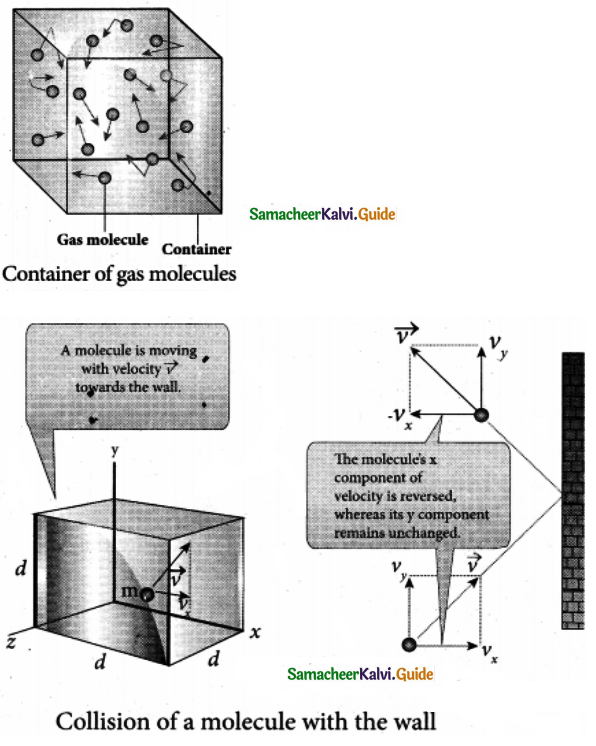The molecules collide with each other and also with the walls of the container. As the collisions are elastic in nature, there is no loss of energy, but a change in momentum occurs.

During each collision, the molecules impart certain momentum, to the wall. Because momentum, is transferred by molecules, the walls experience a continuous force. The force experienced per unit area of the walls of the container determines the pressure exerted by the gas.

Let us consider a molecule of mass m moving with a velocity v having components (vx, vy, vz) hits the right side wall. Since we have assumed that the collision is elastic, the particle rebounds with same speed and its x-component is reversed. The components of velocity of the molecule after collision are (-Vx, Vy, Vz).

The x-component of momentum of the molecule before collision = mvx.
The x-component of momentum of the molecule after collision = – mvx.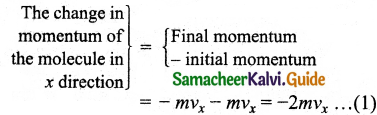According to law of conservation of linear momentum, the change in momentum of the wall = 2 mvx
The molecules within the distance of vx∆t from the right side wall and moving towards the right will hit the wall in the time interval ∆t.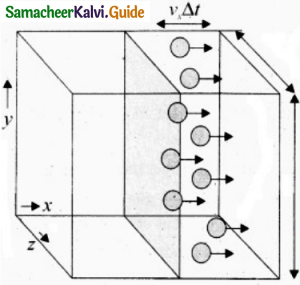The number of molecules that will hit the right side wall in a time interval At is equal to the product of volume (Avx∆t) and number density of the molecules (n). Here A is area of the wall and n is number of molecules per unit volume ($$\frac { N }{ V }$$). It is assumed that the number density is the same throughout the cube.

Not all the n molecules will move to the right, hence on an average only half of then n molecules move to the right and the other half moves towards left side.
The number of molecules that hit the right side wall in a time interval.
∆t = $$\frac { n }{ 2 }$$Avx∆t … (2)
In the same interval of time ∆t, the total momentum transferred by the molecules,
∆p = $$\frac { n }{ 2 }$$Avx∆t x 2mvx
= Av²xmn∆t … (3)
From Newton’s second law, the change in momentum in a small interval of time gives rise to force.
The force exerted by the molecules on the wall is given by
F = $$\frac { ∆p }{ ∆t }$$ = nmAv²x … (4)
Pressure, P = force the area of the wall,
P = $$\frac { F }{ A }$$ = nmv²x… (5)
Since all the molecules are moving completely in random manner, they do not have same speed, so the term v²x can be replaced by the average $$\overline{v_{x}^{2}}$$ in equation (5)
P = nm$$\overline{v_{x}^{2}}$$ … (6)
Since the gas is assumed to move in a random direction, it has no preferred direction of motion. It is implied that the molecule has the same average speed in all three directions. So, $$\overline{v_{x}^{2}}$$ = $$\overline{v_{y}^{2}}$$ = $$\overline{v_{z}^{2}}$$ The mean square speed is obtained from,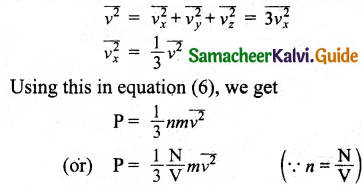Question 3.
Explain in detail the kinetic interpretation of temperature.
We know that,
P = $$\frac { 1 }{ 3 }$$ $$\frac { N }{ V }$$ m$$\overline{v^{2}}$$
PV = $$\frac { 1 }{ 3 }$$ Nm$$\overline{v^{2}}$$ … (1)
Comparing the equation (1) with ideal gas equation PV = NkT,
NkT = $$\frac { 1 }{ 3 }$$ Nm$$\overline{v^{2}}$$
KT = $$\frac { 1 }{ 3 }$$ m$$\overline{v^{2}}$$ … (2)
Multiply the above equation by 3/2 on both sides,
$$\frac { 3 }{ 2 }$$KT = $$\frac { 1 }{ 2 }$$ m$$\overline{v^{2}}$$ … (3)
R.H.S. of the equation (3) is called average kinetic energy of a single molecule ($$\overline{KE}$$).
The average kinetic energy per molecule
$$\overline{KE}$$ = ∈ = $$\frac { 3 }{ 2 }$$KT … (4)
It is implied from equation (3) that the temperature of a gas is a measure of the average translational kinetic energy per molecule of the gas.Question 4.
Describe the total degrees of freedom for monoatomic molecule, diatomic molecule and triatomic molecule.
Monoatomic molecule:
A monoatomic molecule has only three translational degrees of freedom by virtue of its nature.
∴ f = 3
Example: Helium, Neon, Argon.
Diatomic molecule: There are two cases.

(i) At Normal temperature: A molecule of a diatomic gas consists of two atoms bound to each other by a force of attraction, the center of mass lies in the center of the diatomic molecule. So, the motion of the center of mass requires three translational degrees of freedom.
In addition, the diatomic molecule can be rotated about three mutually perpendicular axes.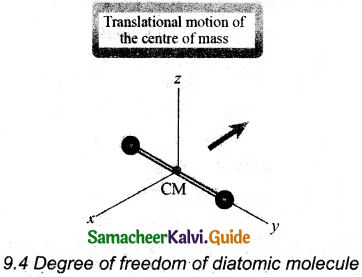In addition, the diatomic molecule can be rotated about three mutually perpendicular axes.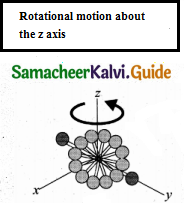But the moment of inertia about its own axis of rotation is negligible. Hence, it has only two rotational degrees of freedom. So totally there are five degrees of freedom.
f = 5

(ii) At high temperature: At a very high temperature such as 5000 K, the diatomic molecules possess additional two degrees of freedom due to vibrational motion [one due to kinetic energy of vibration and the other is due to potential energy].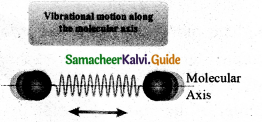So totally there are seven degree of freedom.
f = 7
Example: Hydrogen, Nitrogen, Oxygen.

(i) Linear triatomic molecule:
The linear triatomic molecule has three translational degrees of freedom. It has two rotational degrees of freedom because it is similar to a diatomic molecule except there is an additional atom at the center.

At normal temperature, linear triatomic molecules will have five degrees of freedom. It has two additional vibrational degrees of freedom at high temperatures.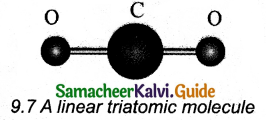So a linear triatomic molecule has seven degrees of freedom.
Example: Carbon dioxide.

(ii) Non-linear triatomic molecule: It has three translational degrees of freedom and three rotational degrees of freedom about three mutually orthogonal axes. So, the total degrees of freedom.
f = 6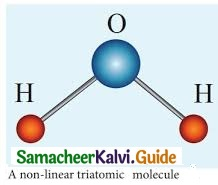Example: Water, Sulphur dioxide.

Question 5.
Derive the ratio of two specific heat capacities of monoatomic, diatomic, and triatomic molecules.
Monoatomic molecule: Average kinetic energy of a molecule
= [$$\frac { 3 }{ 2 }$$kT]
(i) Total energy of a mole of gas
= $$\frac { 3 }{ 2 }$$

(ii) For one mole, the molar specific heat at constant volume
CV = $$\frac { dU }{ dT }$$ = $$\frac { d }{ dT }$$[$$\frac { 3 }{ 2 }$$RT]
CV = $\frac { 3 }{ 2 }$R
Cp = CV + R = $$\frac { 3 }{ 2 }$$R + R
= $$\frac { 5 }{ 2 }$$ R

(iii) The ratio of specific heats,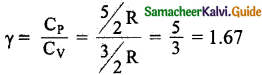Diatomic molecule: Average kinetic energy of a diatomic molecule at low temperature $$\frac { 5 }{ 2 }$$

(i) Total energy of one mole of gas
= $$\frac { 5 }{ 2 }$$kT x NA = $$\frac { 5 }{ 2 }$$RT
(Here, the total energy is purely kinetic)

(ii) For one mole specific heat at constant volume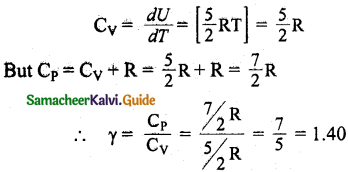(iii) Energy of a diatomic molecule at high temperature is equal to $$\frac { 7 }{ 2 }$$ RT.
CV = $$\frac { dU }{ dT }$$ = [ $$\frac { 7 }{ 2 }$$RT] = [$$\frac { 7 }{ 2 }$$ R
∴CP = CV + R = $$\frac { 7 }{ 2 }$$ R + R
CP = $$\frac { 9 }{ 2 }$$R
It is noted that the CV and CP are higher for diatomic molecules than the monoatomic molecules. It is implied that to increase the temperature of diatomic gas molecules by 1 °C it require more heat energy than monoatomic molecules.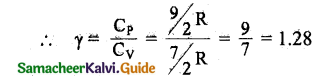Triatomic molecule:
(i) Linear molecule: Energy of one mole
= $$\frac { 7 }{ 2 }$$KT x NA = $$\frac { 7 }{ 2 }$$RT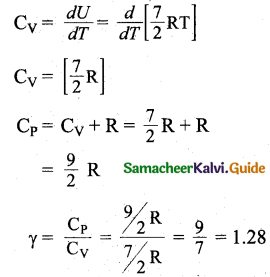(ii) Non-Linear molecule: Energy of one mole
= $$\frac { 6 }{ 2 }$$KT x NA = $$\frac { 6 }{ 2 }$$RT
CV = $$\frac { dU }{ dT }$$ = 3R
CP = CV + R
= 3R + R = 4R
∴ γ = $$\frac{\mathrm{C}_{\mathrm{P}}}{\mathrm{C}_{\mathrm{V}}}$$ = $$\frac { 4R }{ 3R }$$
= $$\frac { 4 }{ 3 }$$
= 1.33

Question 6.
Explain in detail the Maxwell Boltzmann distribution function.
(i) All molecules in any gas move with different velocities in random directions.

(ii) Each molecule collides with every other molecule and their speed is exchanged.

(iii) Let us calculate the rms speed of each molecule and not the speed of each molecule which is rather difficult.

(iv) In general our interest is to find how many gas molecules have the range of speed from v to v + dv.

(v) This is given by Maxwell’s speed distribution function.
Nv = 4πN($$\frac { m }{ 2πkT }$$)3/2 v² $$e^{-m v^{2} / 2 k T}$$
The above expression is graphically shown as follows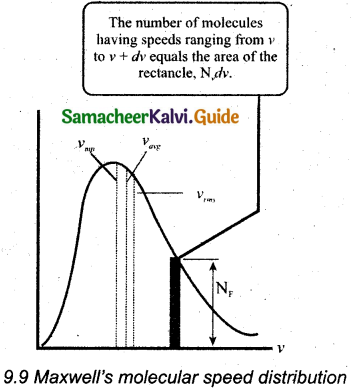(vi) For a given temperature the number of molecules having lower speed increases parabolically but decreases exponentially after reaching most probable speed. The rms speed, average speed, and most probable speed are indicated in the Figure.

(vii) It is found that the rms speed is greatest among the three.

(viii) The area under the graph will give the total number of gas molecules in the system.

(ix) From the speed distribution graph for two different temperatures, it is found that, as temperature increases, the peak of the curve is shifted to the right.

(x) It is implied that the average speed of each molecule will increase. But the area under each graph is the same. Since it represents the total number of gas molecules.Question 7.
Derive the expression for mean free path of the gas.
Let us consider a system of molecules each with diameter d. Let n be the number of molecules per unit volume. It is assumed that only one molecule is in motion and all others are at rest as shown in the figure.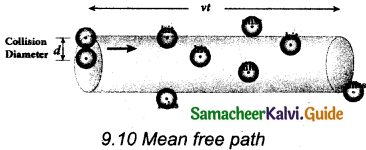If a molecule moves with average speed v in a time t, the distance travelled is vt. In this time t, let us consider the molecule to move in an imaginary cylinder of volume itd2vt. It collides with any molecule whose center is within this cylinder. Hence, the number of collisions is equal to the number of molecules in the volume of the imaginary cylinder. It is equal to πd²vt. The total path length divided by the number of collisions in time t is the mean free path.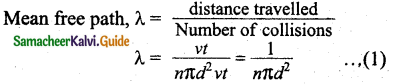It is assumed that only one molecule is moving at a time and other molecules are at rest. But in actual practice all the molecules are in random motion. Hence the average relative speed of one molecule with respect to other molecules has to be taken into account. After some detailed calculations the correct expression for mean free path,
λ = $$\frac{1}{\sqrt{2} n \pi d^{2}}$$
It is implied from the equation that the mean free path is inversely proportional to number density. When the number density increases the molecular collisions increases. Hence it decreases the distance travelled by the molecule before collisions.

Rearranging the equation (2) using ‘m’ (mass of the molecule)
∴ λ = $$\frac{m}{\sqrt{2} \pi d^{2} m n}$$
But mn – mass per unit volume = ρ (density of the gas)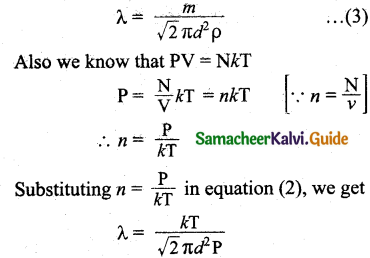Question 8.
Describe the Brownian motion.
The random (Zig – Zag path) motion of pollen suspended in a liquid is called Brownian motion. Brownian motion is due to the bombardment of suspended particles by molecules of the surrounding fluid. Einstein gave the systematic theory of Brownian motion based on kinetic theory and he deduced the average size of molecules.

According to kinetic theory, any particle suspended in a liquid or gas is continuously bombarded from all directions in such a way that the mean free path is almost negligible. This leads to the motion of the particles in a random and zig-zag manner.

Factors affecting Brownian Motion:

• Brownian motion increases with increasing temperature.
• It decreases with bigger particle size, high viscosity, and density of the liquid (or) gas.

IV. Numerical Problems:

Question 1.
Fresh air is composed of nitrogen N2 (78%) and oxygen O2 (21%). Find the rms speed of N2 and O2 at 20°C.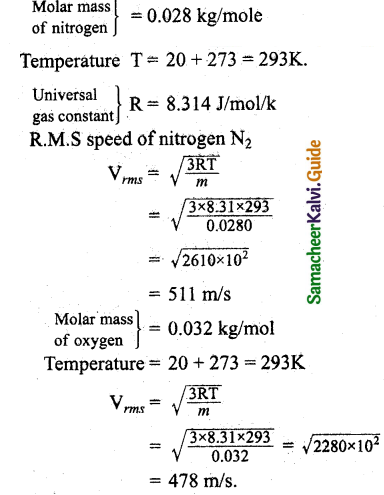Question 2.
If the rms speed of methane gas in Jupiter’s atmosphere is 471.8 ms-1, shows that the surface temperature of Jupiter is sub-zero.
Let the temperature of Jupiter be T.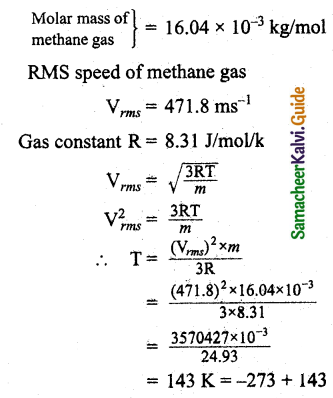The temperature of Jupiter is = -130°CQuestion 3.
Calculate the temperature at which the rms velocity of a gas triples its value at S.T.P.
Let, T = T1 = 273K
RMS velocity,
C = $$\sqrt{\frac{3 \mathrm{RT}_{1}}{\mathrm{M}}}$$ … (1)
When the RMS velocity is tripled,
3C = $$\sqrt{\frac{3 \mathrm{RT}_{2}}{\mathrm{M}}}$$ … (2)
Dividing equation (2) by (1) we get
$$\frac { 3C }{ C }$$ = $$\sqrt{\frac{3 \mathrm{RT}_{2} / \mathrm{M}}{3 \mathrm{R} \times 273 / \mathrm{M}}}$$
3 = $$\sqrt{\frac{\mathrm{T}_{2}}{273}}$$
Squaring on both side
9 = $$\frac{\mathrm{T}_{2}}{273}$$
∴ T2 = 273 x 9 = 2457K
Temperature T2 = 2457K

Question 4.
A gas is at temperature 80°C and pressure 5 x 10-10 Nm-2. What is the number of molecules per m³ if Boltzmann’s constant is 1.38 x 10-23 J k-1.
Temperature T = 80 + 273 = 353K
Pressure P = 5 x 10-10N/m²
KB = $$\frac { R }{ N }$$ ∴ R = KBN
P = nRT = nKBNT
Number of molecules,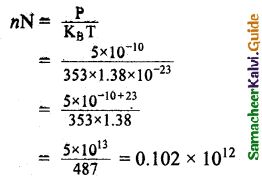Number of molecules = 1.02 x 1011

Question 5.
From kinetic theory of gases, show that Moon cannot have an atmosphere (Assume k = 1.38 x 1023 J K-1, T = 0° C = 273K).
We know that $$\frac { 1 }{ 2 }$$ mV²rms = $$\frac { 3 }{ 2 }$$ kT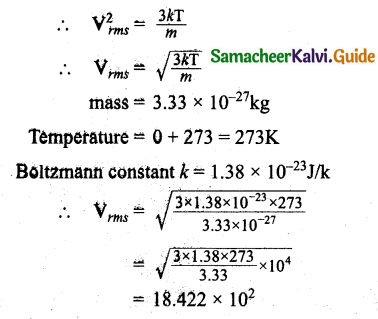Vrms = 1.842 x 10³ ms-1
The value of ve is less than the velocity of gas present on the moon. Hence moon cannot have an atmosphere.

Question 6.
If 1020 oxygen molecules per second strike 4 cm² of the wall at an angle of 30° with the normal when moving at a speed of 2 x 10³ ms-1, find the pressure exerted on the wall, (mass of 1 atom =1.67 x 10-27 kg).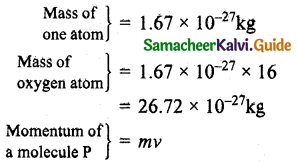Velocity = 2 x 10³ms-1
P = 26.72 x 10-7 x 8 x 2 x 10³
= 427.5 x 10-4 kg ms-1
Component of momentum normal to wall,
PC = P cosθ
Angle θ = 30°
PC = 427.5 x 10-4 cos30°
= 427.5 x 10-4 x $$\frac{\sqrt{3}}{2}$$
= 370.2 x 10-4 kg ms-1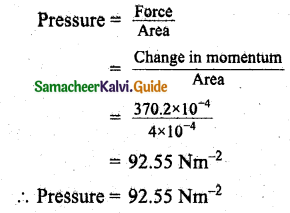Question 7.
During an adiabatic process, the pressure of a mixture of monoatomic and diatomic gases is found to be proportional to the cube of the temperature. Find the value of γ = (CP/CV).
Meyer’s relation is,
CP – CV = R
∴ CP = CV + R
γ = $$\frac{C_{P}}{C_{V}}$$
= $$\frac{C_{V}+R}{C_{V}}$$
γ = 1 + $$\frac{R}{C_{V}}$$
∴ CV = $$\frac { R }{ r-1 }$$
For monoatomic gas, R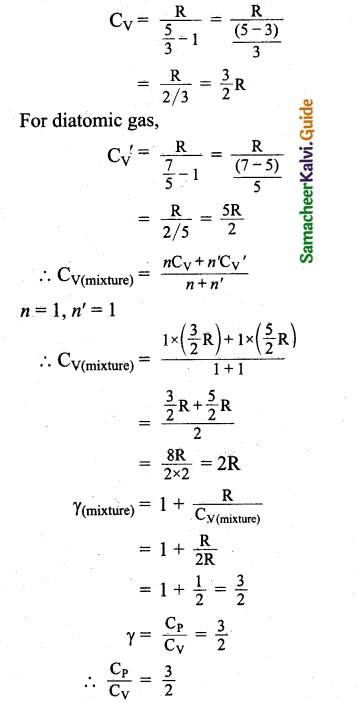Question 8.
Calculate the mean free path of air molecules at STP. The diameter of N2 and O2 is about 3 x 10-10m.
One moles of an ideal gas at S.T.P occupies a volume of 22.4 x 10-3m³.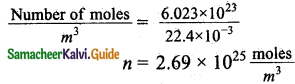In terms of number of molecules and radius the mean free path at S.T.P can be written as,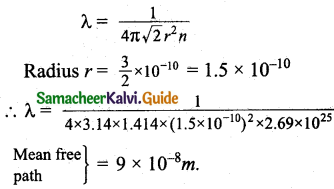Question 9.
A gas made of a mixture of 2 moles of oxygen and 4 moles of argon at temperature T. Calculates the energy of the gas in terms of RT. Neglect the vibrational modes.
Since oxygen is a diatomic molecule with 5 degrees of freedom,
Degree of freedom of molecules in 2 moles of oxygen = f1 = 2N x 5 = 10 N.
Since argon is a monoatomic molecule, degrees of freedom of molecules in 4 moles of argon f2 = 4N x 3 = 12 N.
∴ Total degrees of freedom of the mixture = f = f1 + f2
= 10 N+ 12N
= 22N.
According to the principle of law of equation partition energy, associated with each degree of freedom of a molecule = $$\frac { 1 }{ 2 }$$kT.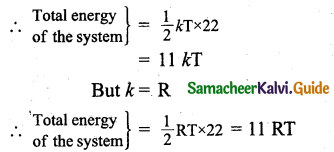Question 10.
Estimate the total number of air molecules in a room of capacity 25 m3 at a temperature of 27°C.
KB = $$\frac { R }{ N }$$
= nN = $$\frac{\mathrm{PV}}{\mathrm{TK}_{\mathrm{B}}}$$
= $$\frac{1.013 \times 10^{5} \times 25}{300 \times 1.38 \times 10^{-23}}$$